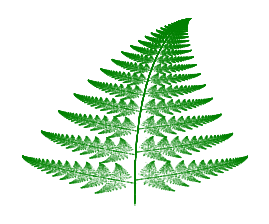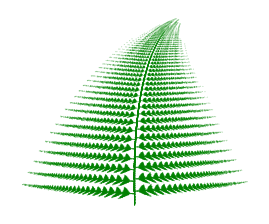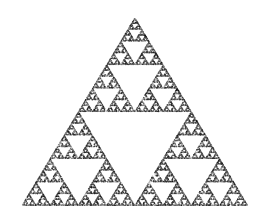﻿ Iterated fractals | TeXblog

## Iterated fractals

I recently dealt with  Iterated function systems , in short IFS. Here we got repeated transformations: the space will be mapped onto itself. There can be different map specifications. We do this an infinite number of timesand take a look at the set in space, which stays invariant. This can be a fractal object.

Enough of theory, there are great books on it, and Wikipedia provides a nice starting point. How do we generate such a fractal image? The simplest approach is the so called chaos game: wie nehmen einen Punkt her, and apply one of the transformations, randomly chosen. Because we got point sets, which are invariant under those transformations, the mapped point will be in the set again. We take the new point and repeat it, thousands of times, until a clear shape appears.

Let’s do this with the famous Barnsley fern!

But how? We need loops and the possibility of calculating affine transformations. It can be done with pgfmath, but I think it’s hardly readable. So I rather take Lua, integrating a programming language in the classical sense into the macro expansion language TeX. It’s easily written in Lua. I put the transformation parameters and probabilities into a matrix, so it can easily be changed for experiments. Let’s start the chaos game!

For compiling, we need LuaTeX and patience. For testing and playing with parameters and probabilities, it’s recommendable to choose a low number of iterations.

\documentclass[tikz,border=10pt]{standalone} \usepackage{luacode} \begin{luacode*} function barnsley(iterations,options) local x = math.random() local y = math.random() local m = { 0.0, 0.0, 0.0, 0.16, 0.0, 0.0, 0.01, 0.85, 0.04, -0.04, 0.85, 0.0, 1.6, 0.85, 0.2, -0.26, 0.23, 0.22, 0.0, 1.6, 0.07, -0.15, 0.28, 0.26, 0.24, 0.0, 0.44, 0.07 } local pm = { m, m + m, m + m + m } if options ~= [[]] then tex.sprint("\\draw[" .. options .. "] ") else tex.sprint("\\addplot coordinates{") end for i=1, iterations do p = math.random() if p < pm then case = 0 elseif p < pm then case = 1 elseif p < pm then case = 2 else case = 3 end newx = (m[7*case+1] * x) + (m[7*case+2] * y) + m[7*case+5] y = (m[7*case+3] * x) + (m[7*case+4] * y) + m[7*case+6] x = newx tex.sprint("("..x..","..y..") circle (0.05pt)") end tex.sprint(";") end \end{luacode*} \begin{document} \begin{tikzpicture} \directlua{barnsley(100000, [[color=green!50!black,fill]])} \end{tikzpicture} \end{document}On TeXwelt.de I produced variations, printed using pgfplots.Also the famous Sierpinski triangle can be generated using the chaos game instead of the L-System approach, the similar source code is on TeXwelt.de, like linked above:Now in three dimensions?No joke – in analogy to the triangle there’s the squarish Sierpinski carpet, wich becomes the so called Menger sponge in three dimensions.

07. July 2014 by stefan